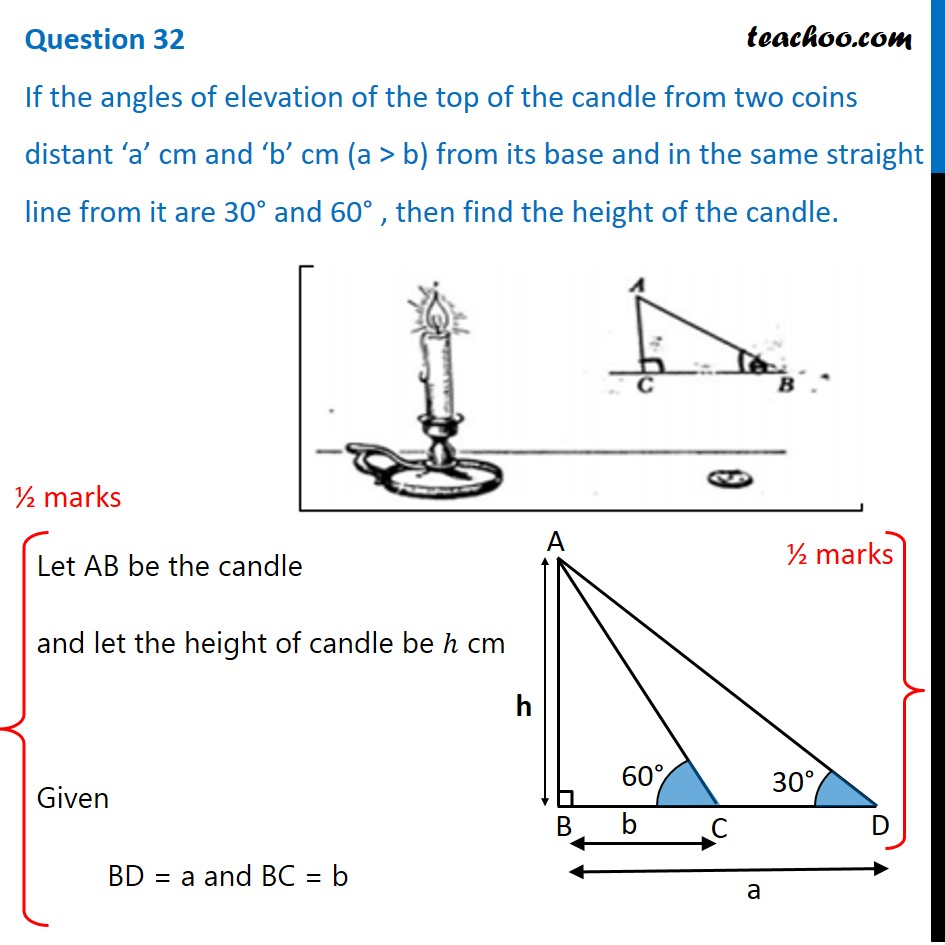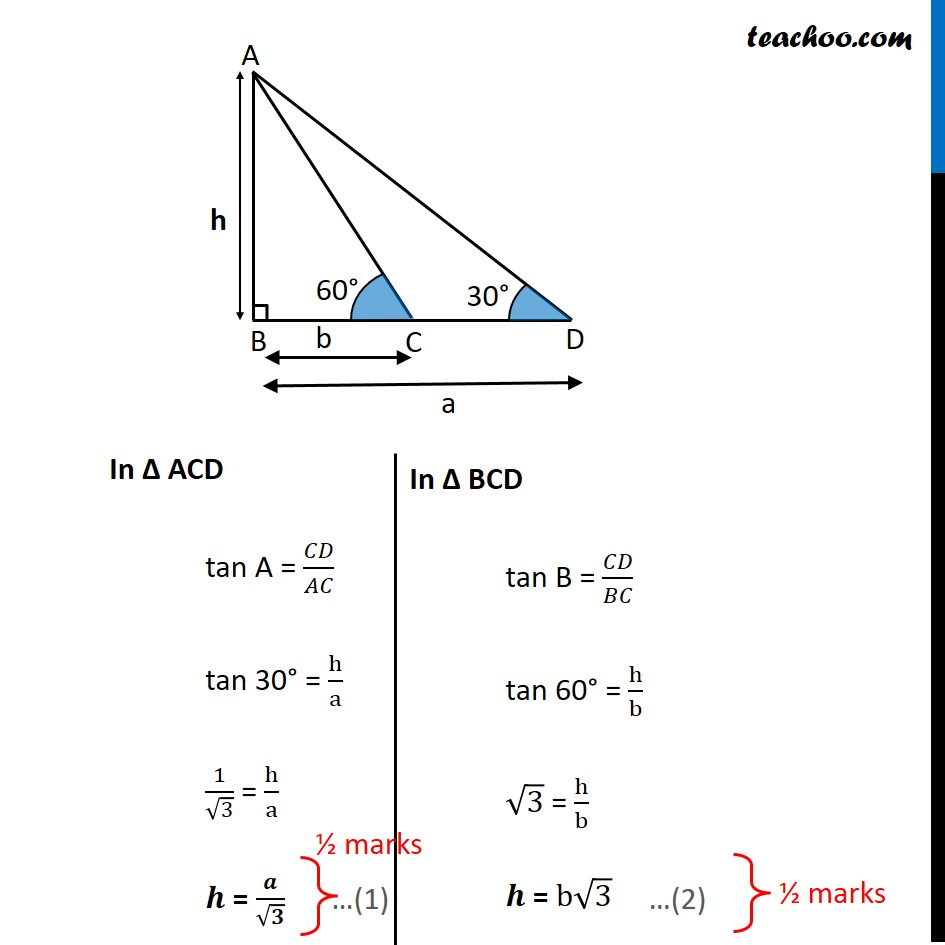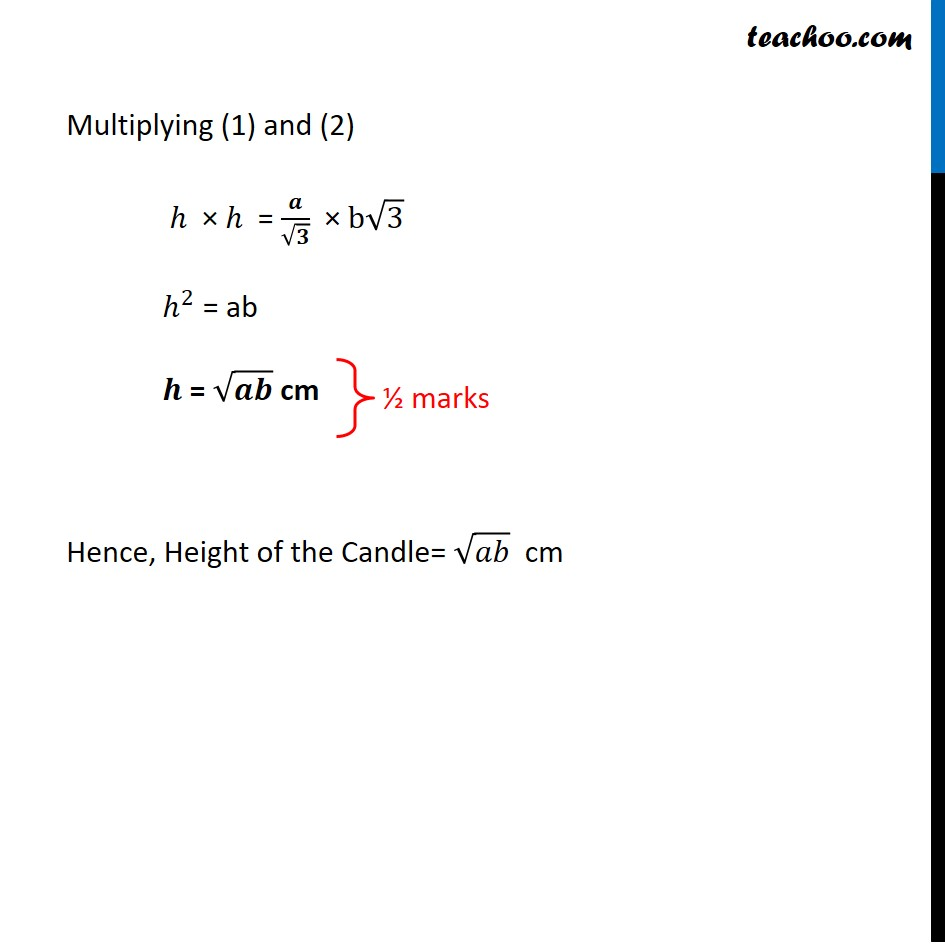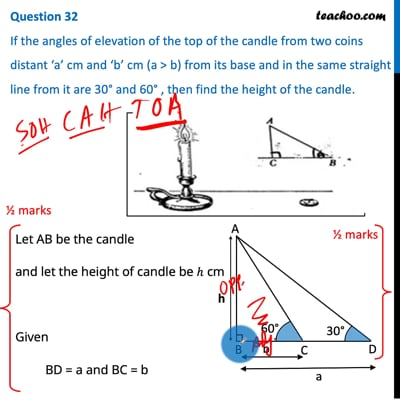CBSE Class 10 Sample Paper for 2021 Boards - Maths Standard

Class 10
Solutions of Sample Papers for Class 10 Boards

## If the angles of elevation of the top of the candle from two coins distant ‘a’ cm and ‘b’ cm (a > b) from its base and in the same straight line from it are 30° and 60° , then find the height of the candle.This video is only available for Teachoo black users

Introducing your new favourite teacher - Teachoo Black, at only ₹83 per month

### Transcript

Question 32 If the angles of elevation of the top of the candle from two coins distant ‘a’ cm and ‘b’ cm (a > b) from its base and in the same straight line from it are 30° and 60° , then find the height of the candle. Let AB be the candle and let the height of candle be ℎ cm Given BD = a and BC = b In Δ ACD tan A = 𝐶𝐷/𝐴𝐶 tan 30° = h/a 1/√3 = h/a 𝒉 = 𝒂/√𝟑 In Δ BCD tan B = 𝐶𝐷/𝐵𝐶 tan 60° = h/b √3 = h/b 𝒉 = b√3 Multiplying (1) and (2) ℎ × ℎ = 𝒂/√𝟑 × b√3 ℎ^2 = ab 𝒉 = √𝒂𝒃 cm Hence, Height of the Candle= √𝑎𝑏 cm